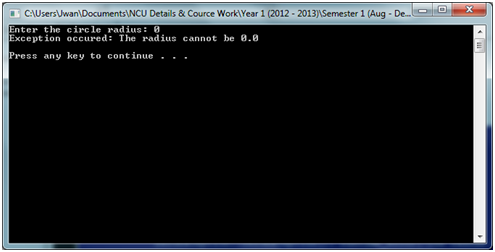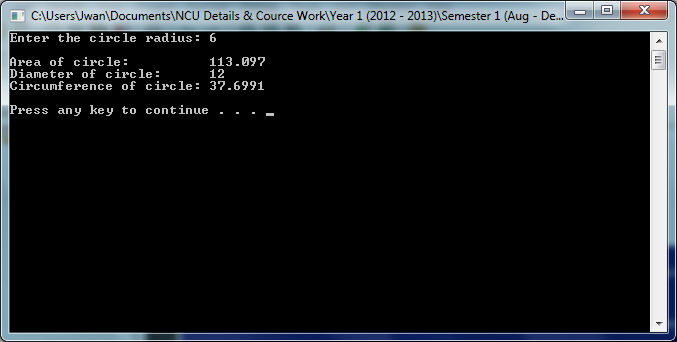Circle Class in C++Posted by Samath Last Updated: January 07, 202124108

Write a Circle class that has the following member variables:

• pi: a double initialized with the value 3.14159

The class should have the following member function:

• Default constructor: A default constructor that sets radius to 1.0

• Constructor: Accepts the radius of the circle as argument. You are required to use exception handling techniques as discussed in class, if a user enters 0.0 for the radius, throw an exception informing the user that the radius cannot be 0.0 and allow them to enter a new value.

• setRadius: A mutator function for the radius variable

• getRadius: An accessor function for the radius variable

• getArea: Returns the area of a circle, which is calculated as area = pi* radius * radius.

• getDiameter: return the diameter of the circle, which is calculated as radius * 2.

• getCircumference: returns the circumference of the circle, which is calculated as circumference = 2 * pi * radius.

You are required to demonstrate your class in a driver program.

Deliverables: Circle.h, Circle.cpp, tester.cpp, ZeroException.h

Output:Circle.cpp

```#include <iostream>
#include <cstdlib>
#include "Circle.h"
#include "ZeroException.h"

using namespace std;

Circle::Circle()
{
}
{
if( radi == 0 || radi == 0.0)
{
ZeroException ex;
}
else
{
}

}

{
if( r == 0 || r == 0.0)
{
ZeroException ex;
}
else
{
}
}

{
}

double Circle::getArea()
{
}

double Circle::getDiameter()
{
return radius * 2;
}

double Circle::getCircumference()
{
return 2 * pi * radius;
}

{
throw 0.0;
}```

Circle.h

```#ifndef CIRCLEH
#define CIRCLEH

#include <iostream>
#include <cstdlib>

using namespace std;

class Circle
{
public:
Circle();

double getArea();

double getDiameter();

double getCircumference();

private:
static const  double pi = 3.14159;
};

#endif```

ZeroException.h

```#ifndef ZeroExceptionH
#define ZeroExceptionH

#include <iostream>
#include <cstdlib>

using namespace std;

class ZeroException
{
public:

};

#endif```

Tester.cpp

```#include <cstdlib>
#include <iostream>
#include "Circle.h"

using namespace std;

int main(int argc, char *argv[])
{

for(;;)
{
try
{
cout<<"Enter the radius: ";
cout<<"Area: "<<cir.getArea()<<endl;
cout<<"Diameter: "<<cir.getDiameter()<<endl;
cout<<"Circumference: "<<cir.getCircumference()<<endl;
break;
}
catch(double e)
{
cout<<"The radius cannot be 0.0"<<endl<<endl;
continue;
}
}

system("PAUSE");
return 0;
}
```I like dis code still... I'm going 2 show u mine#include #include //Library header file contains runtime_error using namespace std; using std::runtime_error; //standard C++ library class runtime_error class ZeroException : public runtime_error //Name of class {       public://Default error message for constructor specifies              ZeroException::ZeroException(): runtime_error("The radius cannot be 0.0")              {                                }                         };good job man!I don't use any break in my code, it just one chance mi give di userThis is di code for the tester.cpp#include #include #include #include "Circle.h" #include "ZeroException.h" using namespace std; int main(int argc, char *argv[]) {     double rad; //Declare variable          cout<<"Enter the circle radius: ";          //Try contain code that might cause exception and code that     //should not execute if exception occur     try     {        cin>>rad; //input value        Circle cork(rad); //instant of the class        cout<yeah i see. the reason y I gave the user another chance is because the question ask me to. if a user enters 0.0 for the radius, throw an exception informing the user that the radius cannot be 0.0 and allow them to enter a new value.